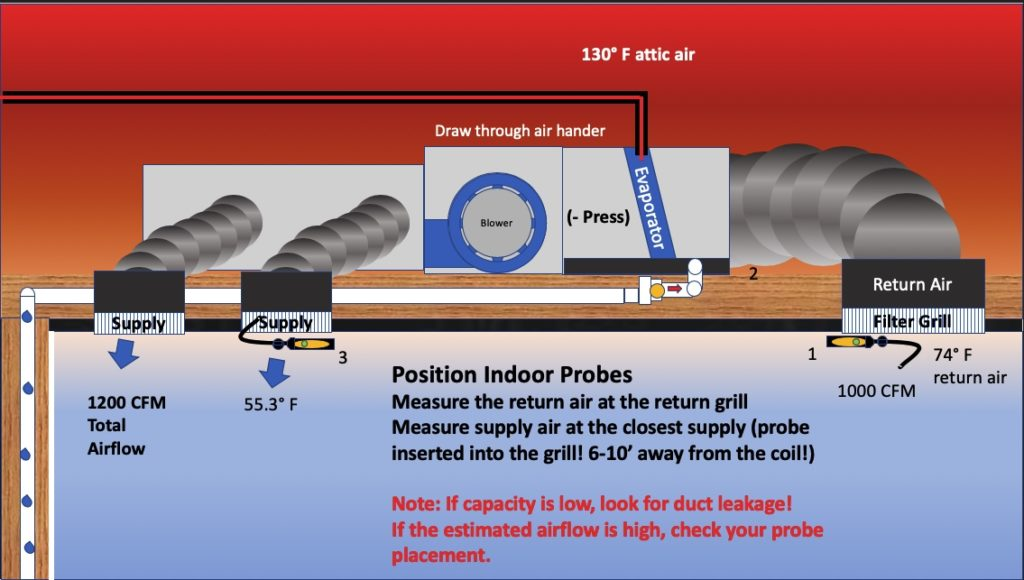# How is the Estimated Airflow Calculated?First, probe placement is critical! insert the supply up into the closest supply register and the return air probe at the face of the return. You need to go into the supply so you do not get a mixed air temperature at the face due to air entrainment. Do not get too close to the evaporator coil either. The probe can be radiant cooled by the coil. Always out of line of site, and 6-10′ away from the coil is ideal. Bad measurements will get you a bad calculation.

So now on to the how to. Well first my apologies, we cannot tell you everything. It is a trade secret that we have refined over the years to account for many variables and conditions. That said, we can share a basic premise and explain what we can do and what we cannot.

It all starts with the fundamental formula Q=mass x specific heat x ΔT

• Q= BTUh
• Mass = lbs of air
• Specific heat of the air =.24 btu/lb
• ΔT = the dry bulb temperature difference

So let’s start with a standard 1 ton system with a standard 400 CFM/ton, and standard air of .075 lbs/Ft³

First to get the mass of the air, 400Ft³/min x .075 lbs/Ft³ =30 Lbs/min of airflow.

Second, let’s solve for ΔT

12,000 = 30 x .24 x Δ T

To get the units consistent we need to multiply the 30 CFM x 60 to get lbs/hr

12,000 = 30 x 60 x .24 x ΔT

12,000/(30 x 60 x .24) = 27.8 degrees

So if the A/C did 100% sensible cooling, we would get a 27.8 degree ΔT

Now we all know that sensible capacity varies with the load, (sensible latent split) and we can get the sensible capacity from the manufacturers extended performance table. A typical sensible latent split would be about .75 so on a 12,000 BTUh unit, 12,000 x .75 = 9000 BTUh sensible and 3000 latent.

So 9000 = (30 x 60 x .24) x ΔT

9000/(30 x 60 x .24 ) = 20.8 or a typical 20 degree split…. That is where the standard 20 ΔT comes from.

So if we know the sensible capacity, and the target ΔT we can calculate the mass of air that is going across the coil. So we can calculate the lbs/min across the coil with a reasonably active evaporator (superheat 0-35 degrees).

9000 = (X x 60 x .24) x 20.8

9000 (20.8 x 60 x .24) = 30.04 lbs/min (remember 30 lbs/min / 400Ft³/min =.075 lbs/Ft³ )

Now to the psychrometric chart….. The inverse of the specific density (.075 lbs/Ft³) is the specific volume (1/.075) = 13.33 Ft³/lb

So once we know the mass flow, we can calculate the volumetric flow per ton of cooling. Knowing that, we simply multiply the airflow/ton by the nominal tons of cooling.

i.e 376 CFM/ton x 5 Tons = 1880 CFM. So while we can calculate the airflow per ton, we cannot determine the volumetric flow rate. We simply have to multiply by the nominal tonnage to get it.

Airflow is typically sufficient at +/- 10% of nominal with a nominal of 350/400/450 CFM/ton.

On the low end, typically we never want to see less than 350 CFM/ton as sensible capacity starts to drop with no gain in latent cooling. In the high end, we typically do not want to see airflow over 475 CFM/ton as the increase in bypass factor negates any additional gains in sensible cooling.

So what is the difference in SCFM (Standard CFM) vs ACFM (Actual CFM)? Standard CFM is what we engineer for. At 400 CFM, it means 30 lbs/min/ton. So as the air density varies, for a typical PSC fan we get a constant airflow with a variable CFM. Meaning we we are moving 2000 boxes of air, but the boxes can vary in weight, so as the air density goes down the mass flow goes down and vice versa.

An ECM works from RPM and torque, so as the mass flow decreases, the torques drops and the ECM speeds up to keep the mass flow the same.

Restated; with an ECM, as the SCFM drop, you will need more ACFM to keep the mass flow rate the same.

400 x .075 = 30 if the air density dropped to .070, you would need 428 ACFM to keep the mass flow rate the same. (30 / .070 = 428 ACFM)

So 400 SCFM = 428 ACFM @ and air density on .070 lbs/Ft³

So while this is not everything, it is enough to let you understand the science behind determining airflow from mass flow, and helps explain one of the mysteries of the beauty of measureQuick.

Updated on March 30, 2021